Question

A charged particle isinjected into a uniform magnetic field such that its velocityvector is perpendicular to themagnetic field vector. Ignoring the particle’s weight, the particlewill

B) move in a straight line.
C) move along a parabolic path.

1.A charged particle is injected into a uniform magnetic field such that its velocity vector is perpendicular to the magnetic field vector. Ignoring the particle’s weight, the particle will follow a circular path.

Option D

Explanation:

Magnetic force causes charged particles to move in spiral paths. The Particle accelerators keep the protons to follow circular paths when it is in the magnetic field. Velocity has a change in direction but magnitude remains the same when this condition exists.

The magnetic force exerted on the charged particle is given by the formula: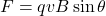where

q is the charge

v is the velocity of the particle

B is the magnetic field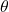is the angle

In this problem, the velocity is perpendicular to the magnetic field vector, hence=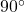and sin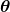=sin 90 degree = 1.

So applying the formula,

the force is simply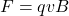Also, the force is perpendicular to both B and v and so according to the right-hand rule, we have:

• a force that is always perpendicular to the velocity, v
• a force which is constant in magnitude (because the magnitude of v or B does not change)

This means that the force acts as a centripetal force, so it will keep the charged particle in a uniform circular motion.